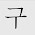## Thursday, 3 July 2014

### Homework: Study Notes (p30) Discussion 2

Describe the various ways that we can use to find out if 3 given points lie on the same straight line.

Key in your responses in "Comments".
Remember to enter your group number.

#### 11 comments:

1.Grp 3

Points lie on the same straight line if either their x or y axis is the same. For example, (5,-1) and (5, 7) lie on the same straight line because the x-axis, 5, is the same for both coordinates.

2.This comment has been removed by the author.

3.There are many ways to see whether the three points are on the same graph.
Say, the three points are (5,-1) , (3,5) , (6,10) and the equation is y=2x-1

Method 1:
We can easily substitute the equation with the coordinates of the points. Therefore, the equation will become
Point A: -1= 2(5)-1 Point B: 5=2(3)-1 Point C: 10= 2(6)-1
-1= 10-1 5=6-1 10= 12-1
-1≠ 9 5=5 10≠ 11
So, only point B is on the graph while A and C are not.

4.Method 2:(the long and tedious method)

You can draw the graph for the equation and plot out those points. Like this, we can see the those points which are not on the graph and are on the graph.

Group 4

5.We can substitute the coordinate value into the equation.

For N: The equation will be -1= -2m+c.
For P : The equation will be 2= 0+c
For Q : The equation will be 5= 2m+c

We can thus conclude that the points do not lie on the same line as they are not the same equations.

6.If either the Y axis and the X axis are the same like (0,2) and (0,5), it means that they are lying on the same line as their Y axis are the same.Or you can plot the graph and see from there.

Group 2

7.Describe the various ways that we can use to find out if 3 given points lie on the same straight line.

If either Y or X axis between them are the same , like (1,8) and (-5 , 8) , for this case , they have the same Y axis so they lie on part of the same line.

Group 4

8.If they have the same gradient and y axis then they are on the same line

Group 1

9.1) If they have exactly the same x or y axis

2) If they have the same gradient

3) If we form and equation using the formula y=mx+c and substitute in the values given,we can find if they are on the same line.

Group 4

10.Either X or Y axis is the same, or using y=mx+c

11.You can use many ways to find if 3 given points lie on the same straight line, I am going to list 2-3
1) You can compare the y-axis/x-axis
--->E.g. Point A =(3,-6) and Point B =(4,-6) This would then mean that these two points are on the same line. This is because they both have the same -6 Coordinate.

2) If they share the same gradient... In other words, these two lines are parallel :D
--->E.g. http://dj1hlxw0wr920.cloudfront.net/userfiles/wyzfiles/0768ac46-e04b-4c5c-9f2b-7748f5196842.gif (its a LINK)

3) Plot the graph then go and see ( Not advised) this method takes alot of time

4) Use the formula y=mx+c Then form an equation

Group 3 :D